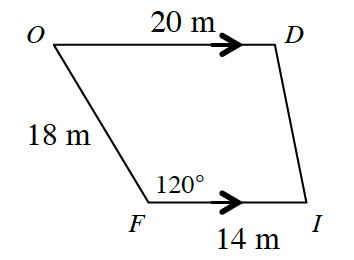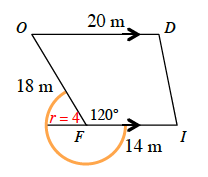### Home > CCG > Chapter 9 > Lesson 9.2.2 > Problem9-81

9-81.The floor plan of Marina’s local drugstore is shown at right. While shopping one day, Marina tied her dog, Mutt, to the building at point $F$. If Mutt’s leash is $4$ meters long, what is the area that Mutt can roam? Draw a diagram and show all work.

$\text{Area of the whole circle}=\pi r^2$. Since $r=4$, the $\text{area}=16\pi$.The area Mutt can roam = (fraction of circle Mutt can roam)(area of entire circle)

$\frac{360^{\circ}-120^{\circ}}{360^{\circ}}=\frac{240^{\circ}}{360^{\circ}}(16\pi)$

Area Mutt can roam $\approx33.51\text{ m}^2$